info@biomedres.us   +1 (502) 904-2126   One Westbrook Corporate Center, Suite 300, Westchester, IL 60154, USA   Site Map

Impact Factor : 0.548

# On Relation of the Universe Mass with a Strong Gravitational Constant Decay Volume 3 - Issue 5

Rostislav F Polishchuk*

• Astro Space Center of the Lebedev, Physics Institute of Russian Academy of Sciences, Russia

Received: April 12, 2018;   Published: April 18, 2018

*Corresponding author: Rostislav F Polishchuk, Astro Space Center of the Lebedev Physics Institute of Russian Academy of Sciences, Leninskii pr. 53, Moscow, 119991 Russia, Email: rpol@asc.rssi

DOI: 10.26717/BJSTR.2018.03.000976

## Abstract

Microphysics is related with cosmology. The Big Bang corresponds to the decay of the unit physical interaction and the decrease of gravitation by 10 in the power of 40, 67. The Universe space curvature radius was growing by the same value: from 103cm to 1028cm. The mass of the Universe matter 2.1056g was growing from the Planck mass by 10 in the power of 61. The total mass with the negative potential mass-energy was zero before and after the Big Bang.

Keywords: Big Bang; De Sitter Space; Strong gravitation in the Past; Planck Mass

## Introduction

The purpose of this paper is to relate the Universe mass with the Planck one after the decay of the unit physical interaction into strong, electroweak and gravitational ones. The Planck length is needed to describe microphysics, while the Newton's gravitational constant is required in cosmology. The connection between those two can easily be illustrated by combining the mass of the Universe 2.1056g with the fundamental physics constants.

Let us revise the value of the fundamental physical constants. Newton's gravitational constant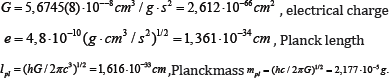Here reduced Planck constant h / 2π and velocity of light c both equal to 1. During the squeeze of the object to its gravitational radius, the get-away speed becomes equal to the speed of light. The object becomes black hole (or white hole in condition that the time sign changes). The body does not collapse into the point with infinite density, because the ultimate density is the Planck one. The asymptotical state of the Universe is the de Sitter space-time as a hyperboloid of one sheet with flat three-dimensional generators and orthogonal time axis sections as three-spheres. To measure the mass of the universe, we shall deem that the capacity of a 3-sphere curvature with radius a together with the matter of critical density will be equal to the next value:Hypothetical Planck particle (planckeon) is the black hole with the Planck mass, with the Planck length (1/2 of its gravitational radius Gmpl | c2 = lpI) and with the limit Planck density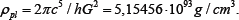Let us introduce the notion of the dimensionless gravitational charge. In the above-mentioned definitions dimensional mass of the planckeon is the reverse Planck length, and the dimensionless mass is 1. The dimensionless mass of any object is m/ mpl.

Now let us consider the following hypothesis: the Planck constant, velocity of light and the dimensionless Planck mass will remain the same during the decay of the unit physical interaction, and the Newton gravitational constant was changed only. It means thatbefore and after the disintegration of the unit physical interaction. After a decrease of the strong original gravitation by 1040,67 times the dimensional Planck mass will increase by 1020,33 times. Therefore, the 3D volume of planckeon before the Big Bang was larger than the modern value by 1061. By multiplying this value on the modern Planck mass, we get the mass of the whole universe. Through the increase of the Planck length by 1061, we have the modern gravitational radius of the Universe [1-3].

This value corresponds to the events horizon in the frame of the observational cosmology. The total matter mass of the Universe is compensated by negative potential energy, hence, the total mass is zero: the boundary of the de Sitter 3-sphere is empty set. Intuitively, we have been used to consider the mass as being imposed into indefinite, flat Minkowski space-time in the spirit of the Island physical system with the Schwarzschild geometry. However, according to Hilbert , actual infinity in the nature does not exist. Homogeneous de Sitter space geometry with equal space points and cosmological term as the source of the curvature is regarded to be more physical. For simplicity, let us compare the mass of the universe with the mass of the mean star, which in our case is the Sun. Nucleons (with their Compton wave length) are the main components of the mass of the Sun. The size of nucleons can be estimated by the formula with gravitational Sun radius and Planck length: 3 . 105cm-1,616 . 10-33cm =(2,2 . 10-14cm)2.

The composition of the Sun's curvature radius with the Planck length gives the size of the star's particle. This size is larger than the Planck length by 1019, while the mass of the particle is smaller than the mass of the planckeon mass by 1019. Planckeon itself is actually the black hole. Consequently, the necessary linear pack 1019 nucleons and cubical pack 1057 nucleons for creating a nucleonic black hole. Through multiplying this number by the mass of nucleon, we have the approximate mass of the Sun.

For the system with radius of events horizon of the Universe, we receive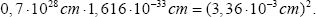We shall name the particle with this size and mass 10-35g as a null particle, zeron. After the decay of matter to de Sitter vacuum, the Universe will consist of 2.1091 zerons. If the origin of the Universe was the de Sitter 3-sphere with mass 2.1056g and Planck density then its curvature radius was 0, 75.10-13cm From the analogical relation 0,75 o 10-13cm o 1,6 o 10-33 cm = (1,1-10-23 cm)2 we receive the particle with the size 1, 1.10-23cm and mass 3, 2.10-15g . We named this particle friedmon [7,8] and we compared exact dual symmetry group SU(2). Exact and broken symmetries and also stable and nonstable particles are change places. The observational cosmology demonstrates the fact of the expanding universe. The speed of the increase of the distances may be endlessly huge and faster than the speed of the light. The expansion began after the Big Bang, 13,7 billion years ago. It is natural to suppose that the original 3-sphere had the limit matter density and the curvature radius 1, 26.10-13cm.

The increase of the curvature radius by 1040,67 (this value is approximate and corresponds to the total mass of the Universe 1061mpl) corresponded to the decrease of the original strong gravitation as the united physical interaction by 1040,67 . After the decay of the united physical interaction the electro-weak interaction was separated into weak and electro- magnetic interactions. The interaction constant depends on the energy. For energies in GeV units 0.01, 0.1, 1 and 100. Strong interaction constant give the consequent values 10, 1, 0.4, 0.12; constants of electro-magnetic interactions: 1/137, 1/135, 1/133, 1/128; constants of weak interactions: 1/26, 1/27, 1/28 and 1/36. The decreasing of the strong interaction constant corresponds to the anti-screening of the strong color charge.

The values, reverse to the constants of interaction, depend logarithmically on the energy and equal to the reverse constant of the interaction α with the following energy value. For the electromagnetic interaction, we used the following formula sin2 θW = 3/8, where the multiplier 3/8 is linked with the weak angle of Weinberg 0W . According to the Theory of Great Unification 1/ αGU = 40 with the energy 1016 GeV. In the experiment we got the value 0.231 for the energy 91 GeV. Under this energy there is a common interaction excluding the gravitation one, which corresponds to a special unitary five dimensional symmetry group.

To define the role of symmetry, we shall assume that the electromagnetic interaction of Lagrangian is invariant to the multiplication of wave function by the unit complex vector. But the Dirac equation for the electron-positron pair remains true in case of the substitution partial derivative for covariant derivative with introduction of potential vector of electromagnetic field, which corresponds to the Maxwell equation. In this sense the electromagnetic field is the gauge, compensating, conserving Dirac equation with including of the electromagnetic interaction. But if imaginary exponents correspond to the one-dimensional commutative symmetry group, then multi-dimensional symmetry group SU(n) is non-commutative. Therefore, the gluons of strong interaction and bozons of weak interaction connected with the groups of symmetry SU (2),SU (3) interact with one another.

However, in the formula SU (2) x U (1) = U (2) the multipliers have different physical significance and are united in a more general group. Intuitively, this shows the inner unity of all physical interactions which are not existent without each other. Symmetries of strong electromagnetic interaction are precise, but the symmetries of weak interaction mix the fermions of different generations, thus, result in the breakage of symmetry and instability of corresponding particles (except the lightest one), broken invariance SU (2) and neutrino-oscillation (periodical change of its types). This was discovered in 1957 by the physician Bruno Pontecorvo (he came once to the Moscow State University and I personally met him, while I was studying in Mechanical, Mathematical Department). Quarks interact by the exchange of gluons, while electrons by the exchange of photons, and both those particles are stable.

The weak interaction is carried out by the change of vector bozons W±,Z° which are instable. The fundamental fermions of this model are six quarks and six leptons. Their interaction is done through the exchange of bozons XY, spin one, with the charges 4e/3, e/3. The strong interactions unite the neutrons with protons in the atom core and are determined by the Yukawa potential U(r) = -(k/r)exp(-r0/r), where k = 1,r0 = 10-13cm. the quantitative theory of strong interaction was formulated in 1935. The measurement of Fermi-moment of helium atom shows that the speed of protons in the atom core equals to the one quarter of light velocity. Therefore, the movement of nucleons in the atom core has a strategically meaning and also increases their movement mass by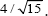.

Nucleons are consists of trio of quarks linked with eight gluons. Quarks have inner quant number named as color, as well as spin, electrical charge and momentum. Nucleon's states are depicted by 3D complex color space. This vector is invariant with respect to these unitary SU (3) groups. It is possible to create colorless combinations from quarks and anti-quarks. Those combinations do not interact with gluons during long-wave approximation. Asymptotical freedom of quarks is created by the anti-screening of their charges. The confinement of quarks happens because they cannot separate from each other longer than 1 fm. They are united with the gluons like a rubber: its stretching means the usage of its mass energy. Therefore, tearing apart this pair will result in a creation of two additional pairs. Hence, we don't observe free quarks. The colorless condition of quark groups called hadrons, which interact with other hadrons (mesons from pairs of quark- antiquarks) connecting hadrons in atom cores. Atoms make up a molecule which participates in a chemical processes which result in restructure of electron layers.

According to Demokritos, the world is made of atoms and emptiness. In chemistry, life is the chemical process with local crowding out of entropy, with self-correction of hereditary code, under the condition of income of free energy. The mind with its understanding and goal-orientation exists as the essential means of saving energy, in comparison with purely instinctive behavior of living organisms. As for the emptiness, it is more everything than nothing. Topological space dimension is 3.The Big Bang and asymptotical condition of the expanding Universe is the two different conditions of a physical vacuum with non-zero density with the cosmological term of the gravitational equations. In this case, the elementary particles are the quantum of the arising vacuum. Quants appear because of the resonance like in the strings. (Non-resonance excitement compensates each other). The physics system action reaches extremum which dictates the appearance of all equations of system dynamics. This way the nature controls its own rules of evolution, and bifurcation creates the world in the shape of the ocean with branching opportunities. Only the possibilities are determined, and a human's choice becomes his responsibility in the light of all determination of behavior in different factors and conditions.

Weak interactions are responsible for beta decay of neutron. Neutrons and protons consist of trios of upper and lower quarks. The decay of neutron creates proton and intermediate electrical charge bozon, which later divides into electron and electronic anti- nitrino. The six types of the different create matrixes of Cabibbo- Kobayashi-Maskava, which are parameterized into three angles and phase multiplier. The theory of Weinberg-Salam electro-weak interaction is the Quantum theory with gauge group. The symmetry is spontaneously broken by the action of Higgs bozon. This bozon was discovered on July 04, 2012 on the Big Hadrons Collider (CERN). Its energy mass is approximately 125 GeV. Its spin, electric and color charges are equal to 1. It is created by the combination of two gluons, charged W- or neutral Z-bozon, which later decay into particle and anti-particle. The particles are photons, b-quark, electron, muon or neutrino.

Higgs bozon make the elementary particles to have their mass of stillness. Since the original value of counter velocity operator is plus-minus the velocity of light only, all particles are created in the form of light with 4-moment of null length. Its square value equals to the subtraction of sum squares of time component and 3-moment: time is the imaginary space coordinate, which is its difference from space dimension. On the quantum level, time may be demonstrated by space series changing each other with Planck frequency per second. This frequency is derived from the combination of Newton's Constant, Planck Constant and the velocity of light. Because of the systematic interaction of the particle with Higgs bozon, 3-moment changes its direction into opposite one. This light reflection of the particle seems like it is remaining on the same spot with null value of its stillness. Weak electro-magnetic interaction originates from the decay of the unified electro-weak interaction, because of the decrease of the interaction energy. Electro-magnetic interaction is caused by the change of electric charges with the virtual photons. These photons are created because of the localization of symmetry u(1) that is because of the introduction of phase ø dependence on space point taken in a single time moment.

The force of electro-magnetic interaction follows the rule of reverse squares. Gravitational interaction also follows this rule. However, the force of electrical interaction is stronger than the gravitational one by 10 in the power of 41. If in the theory of strings the photon spin 1 is a string with open ends, then the graviton which carries gravitational interaction is in fact a closed loop. All elementary particles are the different states of the same particles with different modes of string oscillation. This way, modern physics combines the wholeness and variety of our world. As it was mentioned above, the observational cosmology helped to discover the expansion of the universe (Hubble 1929), theoretically predicted by A.A. Freedman (Saint-Petersburg, 1922). Moreover, in 1918-1920 Freedman used to live with A. Kolchak in Perm city, on the 2nd floor of a small two-stored building. The same building later hosted the book store which became my favorite one. The static equation of physical vacuum (the pressure minus vacuum density) shows that negative pressure causes accelerating recession of sample particles. Galaxies are not sample particles, but they appeared because of the Big Bang when the super-dense vacuum gave all its energy the matter of radiation. The total mass M of the Universe equals 2.1056 which are approximately 100 billion galaxies with 100 billion stars in each galaxy.

The average mass of galaxy is the sum of billions of stars, dark matter and dark energy, the last one increasing the total mass by 20 times. The evolution of the Universe in the beginning corresponded to the expansion of 3-sphere of de Sitter, being originally squeezed to the limit Planck density. The curvature radius of 3-sphere of de Sitter is connected with the mass of the original Universe as in the following equation. The decay of original unit physical interaction into strong electro-weak caused the gravitation getting weaker by 10 in the power of 40, 67. The curvature radius of the Universe increased to the value 40,67 while the density became less by 10 in the power of 122 to reach its critical value. Let us note that the expanding universe is not the black hole, but the white hole, which is derived from the black hole with the change of time sign. To prove this we used de Sitter value, both for the original condition and for the final asymptotical metagalaxy state, when all matter will be decayed, and the vacuum of de Sitter will be created.

De Sitter energy-momentum matter tensor gets equal to the cosmological term. Since the sample particles behind the horizon of events get further from us with the velocity of light, they are not observable. The arcs of their lines are space-like. Strong interaction works only for short distances. Electrical charges of different values compensate each other. The weakest gravitational interaction determines the dynamics of the Universe on the cosmological scale. The Maxwell equation can be written as follows: the co-differential ofthe vector-potential is equal to automatically preserving electrical current. The Einstein equation of gravitation can be written as this: a co-differential of the differential of the tetrad potential equals to the automatically preserving tetrad current . Here the codifferential is covariant divergence form with minus sign, by its first coordinate index. The external differential affects the form in an ordinary way, but with the below anti-symmetrical coordinate indexes. Tetrad-current has coordinate index, which is involved in the Lorentz rotation of the tetrad-field. This field determines the matrix, curvature in Einstein-Cartan theory with Cartan torsion fields which were not in Einstein's General Relativity Theory.

The Einstein-Cartan equations [5,6] are the generalization of the Einstein's ones in the torsion case. This torsion corresponds to the spin of sources. It is necessary to variate the action (the Ricci scalar) by metrics and non-symmetrical connection. Let us Sikj is the matter spin-tensor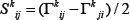and is the torsion tensor of the space-time geometry. Then 16 Einstein-Cartan equations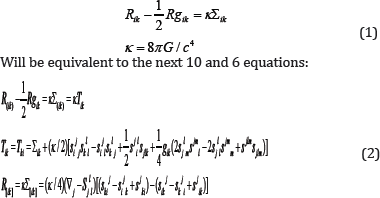Vacuum energy corresponds to 68,9% of the total mass- energy, dark matter 26,8%, atoms 4,9%. This vacuum mass-energy interacts with the visible matter only by gravitation [7,8].